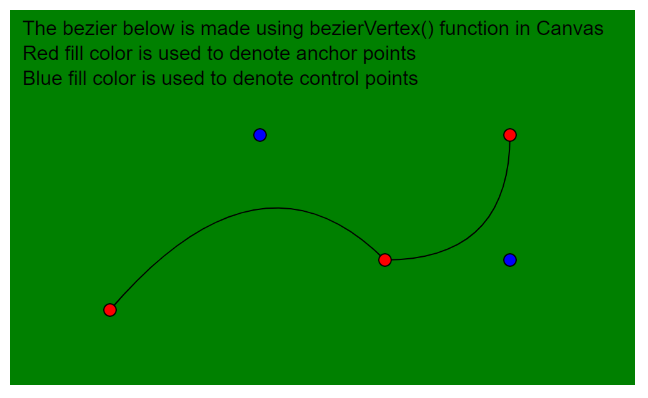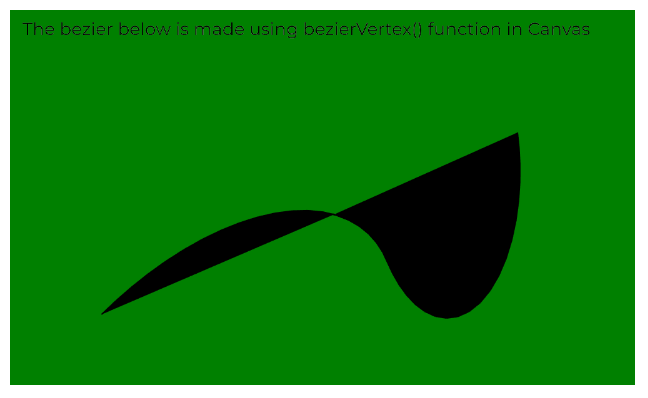# p5.js | quadraticVertex() Function

• Last Updated : 31 May, 2020

The quadraticVertex() function in p5.js is used to specify the vertex coordinates used to draw a quadratic bezier curve. Every call to this function defines the position of one control point and one anchor point of the bezier curve.

This function can only be used between beginShape() and endShape(). It must be prefaced with a call to the vertex() function to set the first anchor point when called with beginShape() for the first time.

The function expects four parameters in 2D mode and six parameters (which includes z coordinates) in 3D mode. Both the 2D and 3D modes can be used for drawing in the WebGL mode.

Syntax:

`quadraticVertex( cx, cy, x3, y3 )`

OR

`quadraticVertex( cx, cy, cz, x3, y3, z3 )`

Parameters: This function accepts six parameters as mentioned above and described below:

• cx: It is a number that specifies the x-coordinate of the control point.
• cy: It is a number that specifies the y-coordinate of the control point.
• x3: It is a number that specifies the x-coordinate of the anchor point.
• y3: It is a number that specifies the y-coordinate of the anchor point.
• cz: It is a number that specifies the z-coordinate of the control point. It is used in the WebGL mode.
• z3: It is a number that specifies the z-coordinate of the anchor point. It is used in the WebGL mode.

The examples below illustrates the quadraticVertex() function in p5.js:

Example 1:

 `function` `setup() { ` `  ``createCanvas(500, 300); ` `  ``textSize(16); ` `} ` ` `  `function` `draw() { ` `  ``background(``"green"``); ` `  ``fill(``"black"``); ` `  ``text( ` `    ``"The bezier below is made using bezierVertex()"``+ ` `    ``" function in Canvas"``, ` `    ``10, ` `    ``20 ` `  ``); ` `  ``text(``"Red fill color is used to denote anchor"``+ ` `       ``" points"``, 10, 40); ` `  ``text(``"Blue fill color is used to denote control"``+ ` `       ``" points"``, 10, 60); ` ` `  `  ``// Define the points ` `  ``// First Anchor Point ` `  ``let p1 = { x: 80, y: 240 }; ` ` `  `  ``// First Control Point ` `  ``let p2 = { x: 200, y: 100 }; ` ` `  `  ``// Second Anchor Point ` `  ``let p3 = { x: 300, y: 200 }; ` ` `  `  ``// Second Control Point ` `  ``let p4 = { x: 400, y: 200 }; ` ` `  `  ``// Third Anchor Point ` `  ``let p5 = { x: 400, y: 100 }; ` ` `  `  ``noFill(); ` ` `  `  ``// Start the quadratic bezier ` `  ``beginShape(); ` ` `  `  ``// Specify the first anchor point ` `  ``// using vertex() ` `  ``vertex(p1.x, p1.y); ` ` `  `  ``// Use the quadraticVertex() function ` `  ``// to define the rest of the points ` `  ``quadraticVertex(p2.x, p2.y, p3.x, p3.y); ` `  ``quadraticVertex(p4.x, p4.y, p5.x, p5.y); ` `  ``endShape(); ` ` `  `  ``// Draw circles for demonstration ` `  ``fill(``"red"``); ` `  ``circle(p1.x, p1.y, 10); ` ` `  `  ``fill(``"blue"``); ` `  ``circle(p2.x, p2.y, 10); ` ` `  `  ``fill(``"red"``); ` `  ``circle(p3.x, p3.y, 10); ` ` `  `  ``fill(``"blue"``); ` `  ``circle(p4.x, p4.y, 10); ` ` `  `  ``fill(``"red"``); ` `  ``circle(p5.x, p5.y, 10); ` `} `

Output:Example 2:

 `let newFont; ` ` `  `function` `preload() { ` `  ``newFont = loadFont(``"fonts/Montserrat.otf"``); ` `} ` ` `  `function` `setup() { ` `  ``createCanvas(500, 300, WEBGL); ` `  ``textFont(newFont, 14); ` `} ` ` `  `function` `draw() { ` `  ``translate(-width / 2, -height / 2); ` ` `  `  ``background(``"green"``); ` `  ``fill(``"black"``); ` `  ``text( ` `    ``"The bezier below is made using bezierVertex()"``+ ` `    ``" function in Canvas"``, ` `    ``10, ` `    ``20 ` `  ``); ` ` `  `  ``// Define the points ` `  ``// First Anchor Point ` `  ``let p1 = { x: 80, y: 240, z: 10 }; ` ` `  `  ``// First Control Point ` `  ``let p2 = { x: 250, y: 100, z: 100 }; ` ` `  `  ``// Second Anchor Point ` `  ``let p3 = { x: 300, y: 200, z: 10 }; ` ` `  `  ``// Second Control Point ` `  ``let p4 = { x: 300, y: 250, z: 200 }; ` ` `  `  ``// Third Anchor Point ` `  ``let p5 = { x: 400, y: 100, z: 10 }; ` ` `  `  ``// Start the quadratic bezier ` `  ``beginShape(); ` ` `  `  ``// Specify the first anchor point ` `  ``// using vertex() ` `  ``vertex(p1.x, p1.y, p1.z); ` ` `  `  ``// Use the quadraticVertex() function ` `  ``// to define the rest of the points ` `  ``quadraticVertex(p2.x, p2.y, p2.z, p3.x, p3.y, p3.z); ` `  ``quadraticVertex(p4.x, p4.y, p4.z, p5.x, p5.y, p5.z); ` `  ``endShape(); ` `} `

Output:Online editor: https://editor.p5js.org/

My Personal Notes arrow_drop_up
Recommended Articles
Page :